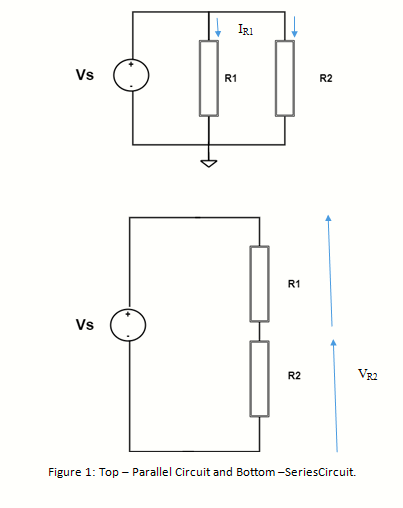Sep 15, 2023

The objective of the Electrical Circuits lab is to expose the students to electrical circuits and give them the experimental skills of lab experiments to continue to build circuit construction skills using different circuit elements. It also aims to introduce MATLAB a circuit simulation software tool.

Parallel Resistors

Build the circuit in Figure 1, Top. Use R1 = 33k ohm and R2 = 18k ohm and 1.5-6 dc V.

Series Resistors

Build the circuit in Figure 1, Bottom. Use R1 = 33k ohm and R2 = 18k ohm and 1.5-6 dc V.Instruction :

1. Calculate
2. Simulate

Measured